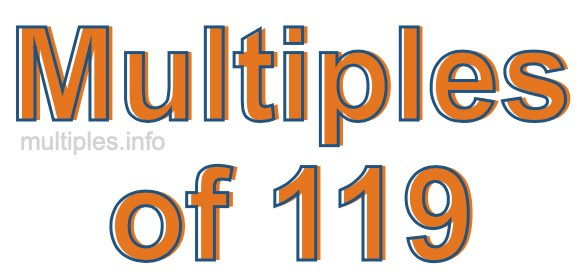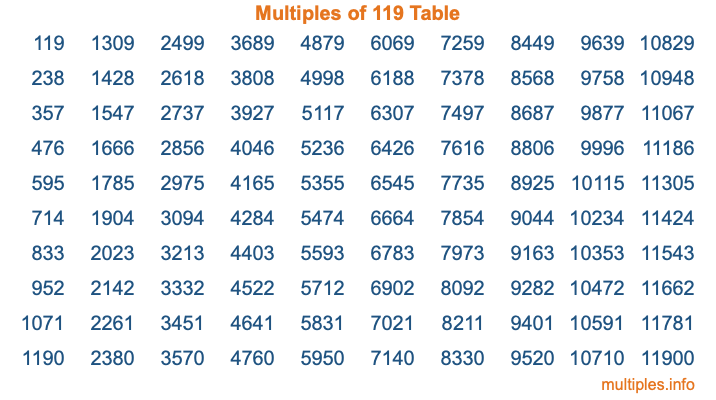Multiples of 119Welcome to the Multiples of 119 page. Here we will first teach you everything you will ever need to know about the multiples of 119, and then give you a study guide summary of everything we taught you to make sure you remember it all. Use this page to look up facts and learn information about the multiples of 119. This page will make you a multiples of one hundred nineteen expert!

Definition of Multiples of 119
Multiples of 119 are all the numbers that when divided by 119 equal an integer. Each of the multiples of 119 are called a multiple. A multiple of 119 is created by multiplying 119 by an integer.

Therefore, to create a list of multiples of 119, you start with 1 multiplied by 119, then 2 multiplied by 119, then 3 multiplied by 119, and so on for as long as you want. Thus, the list of the first five multiples of 119 is 119, 238, 357, 476, and 595. To see a larger list of multiples of 119, see the printable image of Multiples of 119 further down on this page. We also have a category where you can choose any nth multiple of 119.

Multiples of 119 Checker
The Multiples of 119 Checker below checks to see if any number of your choice is a multiple of 119. In other words, it checks to see if there is any number (integer) that when multiplied by 119 will equal your number. To do that, we divide your number by 119. If the the quotient is an integer, then your number is a multiple of 119.

Is  a multiple of 119?

Least Common Multiple of 119 and ...
A Least Common Multiple (LCM) is the lowest multiple that two or more numbers have in common. This is also called the smallest common multiple or lowest common multiple and is useful to know when you are adding our subtracting fractions. Enter one or more numbers below (119 is already entered) to find the LCM.

Check out our LCM Calculator if you need more details about the Least Common Multiple or if you need the LCM for different numbers for adding and subtraction fractions.

nth Multiple of 119
As we stated above, 119 is the first multiple of 119, 238 is the second multiple of 119, 357 is the third multiple of 119, and so on. Enter a number below to find the nth multiple of 119.

th multiple of 119

Multiples of 119 vs Factors of 119
119 is a multiple of 119 and a factor of 119, but that is where the similarities end. All postive multiples of 119 are 119 or greater than 119. All positive factors of 119 are 119 or less than 119.

Below is the beginning list of multiples of 119 and the factors of 119 so you can compare:

Multiples of 119: 119, 238, 357, 476, 595, etc.

Factors of 119: 1, 7, 17, 119

As you can see, the multiples of 119 are all the numbers that you can divide by 119 to get a whole number. The factors of 119, on the other hand, are all the whole numbers that you can multiply by another whole number to get 119.

It's also interesting to note that if a number (x) is a factor of 119, then 119 will also be a multiple of that number (x).

Multiples of 119 vs Divisors of 119
The divisors of 119 are all the integers that 119 can be divided by evenly. Below is a list of the divisors of 119.

Divisors of 119: 1, 7, 17, 119

The interesting thing to note here is that if you take any multiple of 119 and divide it by a divisor of 119, you will see that the quotient is an integer.

Multiples of 119 Table
Below is an image of the first 100 multiples of 119 in a table. The table is in chronological order, column by column. The first column has the first ten multiples of 119, the second column has the next ten multiples of 119, and so on.The Multiples of 119 Table is also referred to as the 119 Times Table or Times Table of 119. You are welcome to print out our table for your studies.

Negative Multiples of 119
Although not often discussed or needed in math, it is worth mentioning that you can make a list of negative multiples of 119 by multiplying 119 by -1, then by -2, then by -3, and so on, to get the following list of negative multiples of 119:

-119, -238, -357, -476, -595, etc.

Multiples of 119 Summary
Below is a summary of important Multiples of 119 facts that we have discussed on this page. To retain the knowledge on this page, we recommend that you read through the summary and explain to yourself or a study partner why they hold true.

There are an infinite number of multiples of 119.

A multiple of 119 divided by 119 will equal a whole number.

119 divided by a factor of 119 equals a divisor of 119.

The nth multiple of 119 is n times 119.

The largest factor of 119 is equal to the first positive multiple of 119.

119 is a multiple of every factor of 119.

119 is a multiple of 119.

A multiple of 119 divided by a divisor of 119 equals an integer.

119 divided by a divisor of 119 equals a factor of 119.

Any integer times 119 will equal a multiple of 119.

Multiples of a Number
Here you can get the multiples of another number, all with the same attention to detail as we did for multiples of 119 on this page.

Multiples of
Multiples of 120
Did you find our page about multiples of one hundred nineteen educational? Do you want more knowledge? Check out the multiples of the next number on our list!